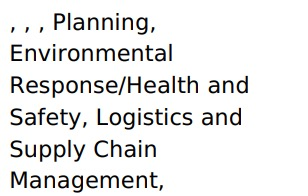# Concat Equation - Hide comma and space when empty field/string

Greetings Community-

Can anyone help with an equation…I seem to be stuck…

I’d like to concatenate several fields in a table and separate with a comma and space. However, when a field is empty/NULL, I’d like to hide the comma and space.

Current Equation:

``````CONCAT({Prevent Cap Txt Formula},CHAR(44),CHAR(32),{Protect Cap Txt Formula},CHAR(44),CHAR(32),{Mitigate Cap Txt Formula},CHAR(44),CHAR(32),{Response Cap Txt Formula},CHAR(44),CHAR(32),{Recovery Cap Txt Formula})
``````

Example when field is emptyWhen I tried Iris AI it gave me a formula of:

``````CONCAT({Prevent Cap Txt Formula}, IF(NOT({Protect Cap Txt Formula} = ''),CONCAT(', ', {Protect Cap Txt Formula}),''), IF(NOT({Mitigate Cap Txt Formula} = ''), CONCAT(', ', {Mitigate Cap Txt Formula}),''), IF(NOT({Mitigate Cap Txt Formula} = ''), CONCAT(', ', {Mitigate Cap Txt Formula}),''), IF(NOT({Response Cap Txt Formula} = ''),CONCAT(', ', {Response Cap Txt Formula}),''), IF(NOT({Recovery Cap Txt Formula} = ''),CONCAT(', ', {Recovery Cap Txt Formula}),''))
``````

However, “NOT” is not a valid function in Tadabase.

Any help would be appreciated!